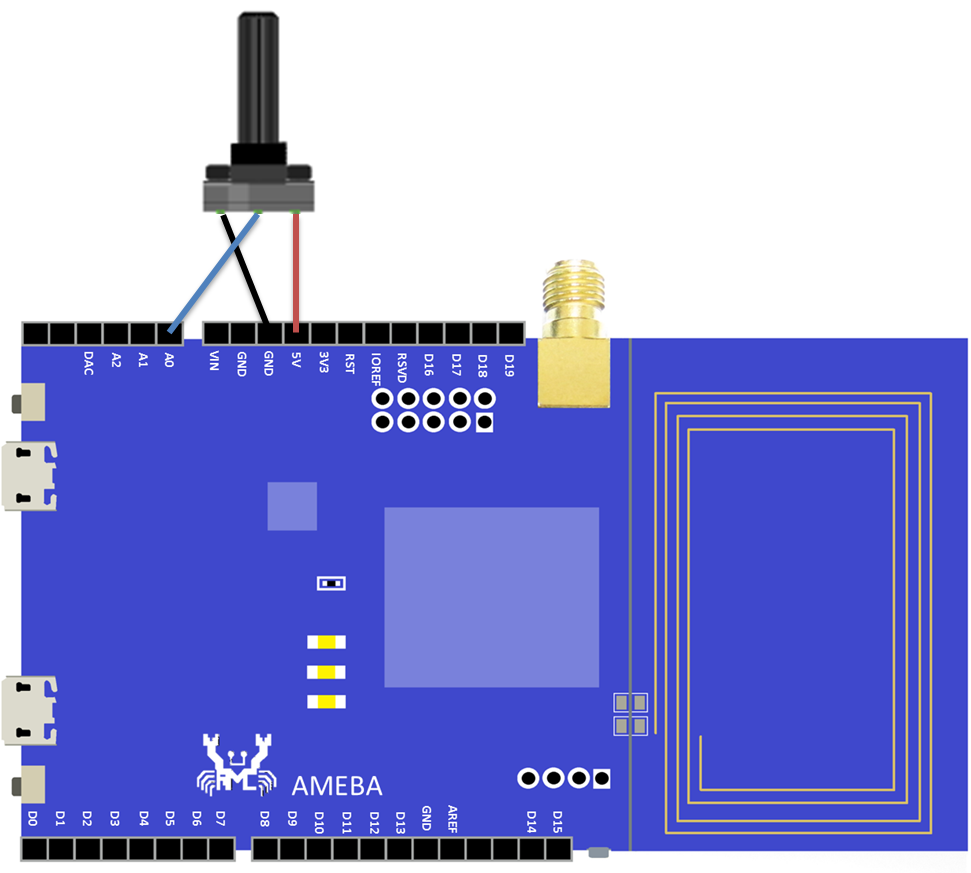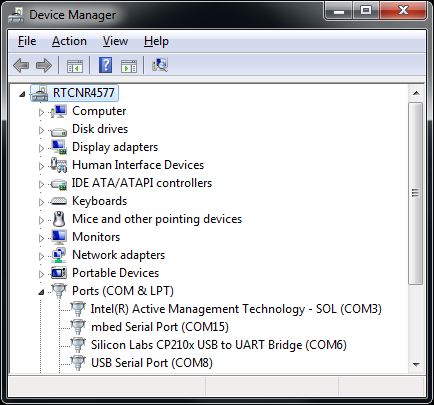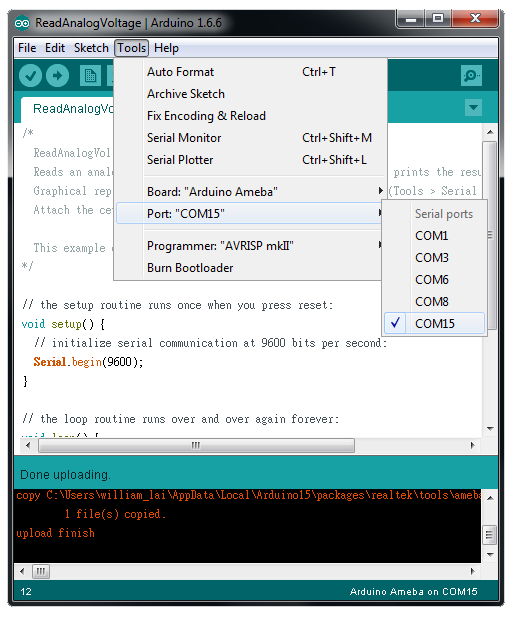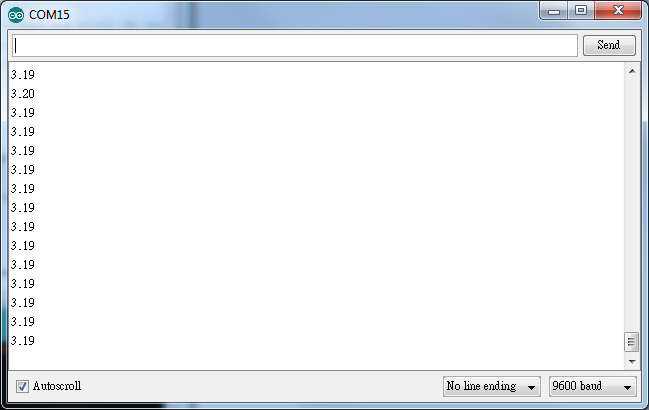Ameba Arduino: [RTL8195AM] ADC - Use ADC and Ameba to make a voltmeter

• ADC (Analog to Digital Converter) component is capable of coverting analog signal to digital signal. The ADC component of Ameba is of 12-bit precision, and its effective range of measurement is 0V ~ 3.3V, so the minimum measurable difference i voltage is 3.3V / (2^12-1) = 8mV.
Materials
• Ameba x 1
• 10KΩ Potentiometer (Variable Resistor) x 1, if you don’t have potentiometer in hand, the 3V3 & 5V on Ameba can be used instead.
Example
ADC is widely used in processing analog signal, such as voltage, audio signal, light, temperature, …etc. In real-life use case, ADC can be used to measure the voltage of a battery, or read the value of a volume knob.
In this example, we use ADC to get the voltage value. Open the sample code in “File” -> “Examples” -> “01.Basics” -> “ReadAnalogVoltage” There are 3 ADC pins on Ameba (A0~A2), we will use A0 in this example. First, connect the output of the potentiometer to A0, then connect VDD of the potentiometer to GND as the figure:To read data from Ameba, we have to find out the serial port connected to Ameba. Open “Device Manager” in Windows, find “mbed Serial Port”. For example in the figure, we can find “mbed Serial Port(COM15)”, then “COM15” is the port number connected to Ameba.Next, we connect Ameba to the correct port in Arduino IDE. Go to “Tools” -> “Port”, and select the port number (here we select port COM15).When we want to read the message output from Ameba, it is necessary that the port is set to the correct port connecting to Ameba:Then we open the “Serial Monitor” in “Tools” -> “Serial Monitor”: In the Serial Monitor, Ameba prints the voltage value output from A0 continuously. We can adjust the potentiometer to change the output.If you don’t have potentiometer in hand, you can connect 3V3 and A0, and the output value would be 3.3V approximately.
Code Reference
You can find detailed information of this example in the documentation of Arduino: https://www.arduino.cc/en/Tutorial/ReadAnalogVoltage We use analogRead(pin) to read the value from the specified analog pin directly: https://www.arduino.cc/en/Reference/AnalogRead

IoT SoC / MCU
Video SoC

Forums

Forums